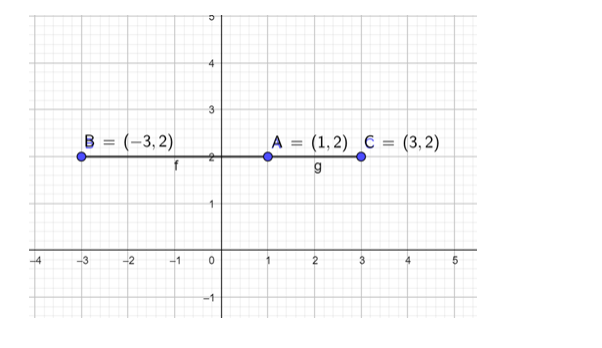# If A(1, 2), B(-3, 2) and C(3, 2) be the vertices of a ΔABC, show thatQuestion:

If $A(1,2), B(-3,2)$ and $C(3,2)$ be the vertices of a $\triangle A B C$, show that

(i) $\tan \mathrm{A}=2$

(ii) $\tan \mathrm{B}=\frac{2}{3}$

(iii) $\tan \mathrm{C}=\frac{4}{7}$

Solution:

Points A,B,C lie on a same line, therefore the slope of each line is same and hence it does not form a triangle.